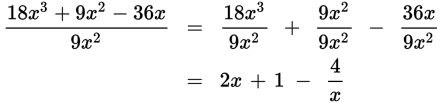# SAT Math Multiple Choice Question 332: Answer and Explanation

### Test Information

Question: 332

2.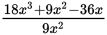Which of the following is equivalent to the expression above?

• A.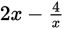• B.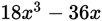• C.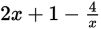• D.Explanation:

C

Difficulty: Easy

Category: Passport to Advanced Math / Exponents

Strategic Advice: Don't be tempted-you can't simply cancel one term when a polynomial is divided by a monomial. You can, however, split the expression into three terms, each with a denominator of 9x 2, and simplify. You could also use polynomial long division to answer the question. Use whichever method gets you to the answer more quickly on Test Day.